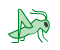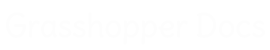﻿ Grasshopper Maths - Addon for Grasshopper | Grasshopper DocsCommunity documentation for Grasshopper add-ons & plugins# Grasshopper Maths

##### SYSTEM COMPONENTS. Version 1.0.0007.Released on 2022-Mar-08.Provides 102 components.Created by David Rutten.Features 0 video tutorials.
 Domain
 Matrix
 Operators
 Polynomials
 Script
 Time
 Trig
 Util

### Operators

 Gate And (And)Perform boolean conjunction (AND gate). Gate Majority (Vote)Calculates the majority vote among three booleans. Equality (Equals)Test for (in)equality of two numbers Factorial (Fac)Returns the factorial of an integer. Larger Than (Larger)Larger than (or equal to) Mass Addition (MA)Perform mass addition of a list of items Mass Multiplication (MM)Perform mass multiplication of a list of items Gate Nand (Nand)Perform boolean alternative denial (NAND gate). Gate Nor (Nor)Perform boolean joint denial (NOR gate). Gate Not (Not)Perform boolean negation (NOT gate). Gate Or (Or)Perform boolean disjunction (OR gate). Relative Differences (RelDif)Compute relative differences for a list of data Similarity (Similar)Test for similarity of two numbers Smaller Than (Smaller)Smaller than (or equal to) Addition (A+B)Mathematical addition Multiplication (A×B)Mathematical multiplication Subtraction (A-B)Mathematical subtraction Gate Xnor (Xnor)Perform boolean biconditional (XNOR gate). Gate Xor (Xor)Perform boolean exclusive disjunction (XOR gate). Division (A/B)Mathematical division Integer Division (A\B)Mathematical integer division Modulus (Mod)Divides two numbers and returns only the remainder. Power (Pow)Raise a value to a power. Absolute (Abs)Compute the absolute of a value. Negative (Neg)Compute the negative of a value.

### Util

 Average (Avr)Solve the arithmetic average for a set of items Blur Numbers (NBlur)Blur a list of numbers by averaging neighbours Complex Argument (Arg)Get the argument of a Complex number Complex Components (Complex)Extract the Real and Imaginary components from a complex number Complex Conjugate (z*)Create the conjugate of a Complex number Complex Modulus (CMod)Get the modulus of a Complex number Create Complex (Complex)Create a complex number from a Real and an Imaginary component Natural logarithm (E)Returns a factor of the natural number (e). Epsilon (Eps)Returns a factor of double precision floating point epsilon. Extremes (Extrz)Find the extremes in a list of values Interpolate data (Interp)Interpolate a collection of data. Golden Ratio (Phi)Returns a factor of the golden ratio (Phi). PiReturns a factor of Pi. Truncate (Trunc)Perform truncation of numerical extremes Weighted Average (Wav)Solve the arithmetic weighted average for a set of items RoundRound a floating point value. Maximum (Max)Return the greater of two items. Minimum (Min)Return the lesser of two items.

### Trig

 ArcCosine (ACos)Compute the angle whose cosine is the specified value. ArcSine (ASin)Compute the angle whose sine is the specified value. ArcTangent (ATan)Compute the angle whose tangent is the specified value. Cosine (Cos)Compute the cosine of a value CoSecant (Csc)Compute the co-secant (reciprocal of the Sine) of an angle. CoTangent (Cot)Compute the co-tangent (reciprocal of the Tangent) of an angle. Secant (Sec)Compute the secant (reciprocal of the Cosine) of an angle. Sine (Sin)Compute the sine of a value SincCompute the sinc (Sinus Cardinalis) of a value. Tangent (Tan)Compute the tangent of a value Degrees (Deg)Convert an angle specified in radians to degrees Radians (Rad)Convert an angle specified in degrees to radians Right Trigonometry (RTrig)Right triangle trigonometry CentroidGenerate the triangle centroid from medians. Circumcentre (CCentre)Generate the triangle circumcentre from perpendicular bisectors. Incentre (ICentre)Generate the triangle incentre from angle bisectors. Orthocentre (OCentre)Generate the triangle orthocentre from altitudes. Triangle Trigonometry (Trig)Generic triangle trigonometry

### Domain

 Construct Domain² (Dom²)Create a two-dimensional domain from two simple domains. Construct Domain (Dom)Create a numeric domain from two numeric extremes. Deconstruct Domain² (DeDom2)Deconstruct a two-dimensional domain into its component parts Deconstruct Domain (DeDomain)Deconstruct a numeric domain into its component parts. Divide Domain² (Divide)Divides a two-dimensional domain into equal segments. Divide Domain (Div)Divide a domain into equal segments. Find Domain (FDom)Find the first domain that contains a specific value Includes (Inc)Test a numeric value to see if it is included in the domain Consecutive Domains (Consec)Create consecutive domains from a list of numbers Bounds (Bnd)Create a numeric domain which encompasses a list of numbers. Bounds 2D (Bnd)Create a numeric two-dimensional domain which encompasses a list of coordinates. Remap Numbers (ReMap)Remap numbers into a new numeric domain

### Polynomials

 Log N (LogN)Return the N-base logarithm of a number. Power of 10 (10º)Raise 10 to the power of N. Power of 2 (2º)Raise 2 to the power of N. CubeCompute the cube of a value Cube Root (Cbrt)Compute the cube root of a value Power of E (Eº)Raise E to the power of N. Logarithm (Log)Compute the Base-10 logarithm of a value. One Over X (1/x)Compute one over x. Square (Sqr)Compute the square of a value Square Root (Sqrt)Compute the square root of a value

### Time

 Combine Date & Time (CDate)Combine a pure date and a pure time into a single date Construct Date (Date)Construct a date and time instance. Construct Exotic Date (DateEx)Construct a date using a specific calendar Construct Smooth Time (SmTime)Construct a time instance from smooth components Construct Time (Time)Construct a time instance Date Range (RDate)Create a range of successive dates or times Deconstruct Date (DDate)Deconstruct a date into years, months, days, hours, minutes and seconds Interpolate Date (IntDate)Interpolate between two dates or times.

### Matrix

 Construct Matrix (Matrix)Construct a matrix from initial values Deconstruct Matrix (DeMatrix)Deconstruct a matrix into its component parts Invert Matrix (MInvert)Invert a matrix Swap Columns (SwapC)Swap two columns in a matrix Swap Rows (SwapR)Swap two rows in a matrix Transpose Matrix (Transpose)Transpose a matrix (swap rows and columns)

### Script

 GhPython Script (Python)GhPython provides a Python script component Evaluate (Eval)Evaluate an expression with a flexible number of variables. ExpressionEvaluate an expression C# Script (C#)A C#.NET scriptable component VB Script (VB)A VB.NET scriptable component

Site design © Robin Rodricks.   Grasshopper Maths and associated data © 2022 Robert McNeel & Associates.
Rhinoceros and Grasshopper are registered trademarks of Robert McNeel & Associates.  Hosted by GitHub